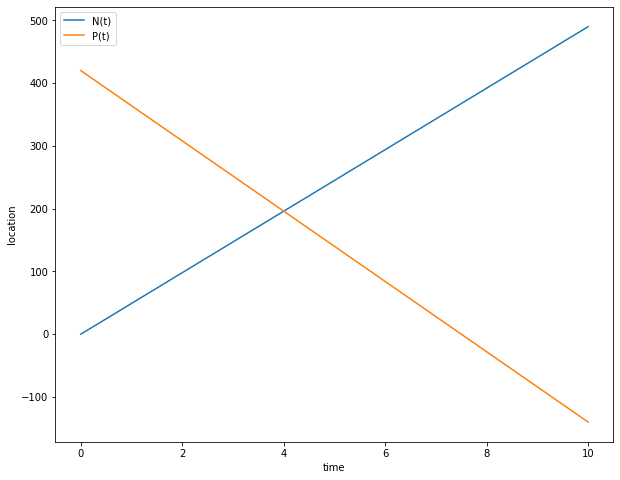# Elementary mathematics tutorials¶

By Max Kapur. Updated Nov. 10, 2019.

In :
import numpy as np
from matplotlib.pyplot import plot, legend, xlabel, ylabel, figure


## A typical standardized-test problem¶

This problem was sent to me by GRE George, a friend preparing for the infamous general-purpose graduate-school entrance exam. The explanation given in his practice book was rather terse, so I wrote him a more detailed one.

City A and City B are connected by a straight 420-mile road. At noon, Nigel left City A, travling toward City B, and Phyllis left City B, traveling toward City A. If Nigel travels at 49 miles per hour and Phyllis travels at 56 miles per hour, how many miles apart will Nigel and Phyllis be 1 hour before they meet?

There are really two unknowns in this problem:

1. At what time will Nigel and Phyllis meet?
2. How far apart are Nigel and Phyllis an hour before that time?

Let’s work with $$ first. George’s textbook probably wants him to set up a “coordinate system.” In this case, that means a line representing the 420-mile road. At point $(0)$, we have City A, and at point $(420)$ we have City B.

Since Nigel and Phyllis both leave at noon, let’s just call noon time $0$ and create an equation that gives each of their locations $t$ hours after noon.

Nigel is going from A to B. That means his position along the line is going to increase at the rate of 49 mph. So,

$$N(t) = 49t$$

where $t$ represents the hours since noon and $N(t)$ represents his position.

(Note that $N(t)$ means “$N$, a function of $t$” not “$N$ times $t$,” just like how $f(x)$ doesn’t mean “f times x.”)

Phyllis is going to A from B. Since she’s traveling in the opposite direction as Nigel, her speed is actually negative 56 mph. She starts at position $420$ (where City B is) and loses 56 miles for every hour past noon. So,

$$P(t) = 420 - 56t$$

Now, to answer $$, all we need to do is figure out the time $t$ at which the functions $N(t)$ and $P(t)$ intersect. Since one function is going up from zero, and the other function is going down from 420, we can be sure that they will intersect somewhere. To find that point, all we need to do is set $N(t)$ and $P(t)$ equal to each other and solve for $t$.

That’s just algebra:

$$420 - 56t = 49t$$

Add $56t$ to both sides:

$$420 = 105t$$

Divide by 105:

$$4 = t$$

Looks like they should meet after four hours. Here’s a graph, just to make double sure. You can see that the lines cross at $t = 4$. Before you look: How far apart are Nigel and Phyllis now?

(Answer: Now, at $t = 4$, they are zero miles apart. The problem asks us how far apart they are an hour before this, which is what we’ll do next.)

In :
def N(t): return 49*t
def P(t): return 420 - 56*t
t= np.linspace(0,10,51)

figure(figsize=(10,8))
plot(t,N(t),label='N(t)')
plot(t,P(t),label='P(t)')

legend()
xlabel('time')
ylabel('location')

Out:
Text(0, 0.5, 'location')OK, now let’s answer $$. An hour before $t = 4$ is $t = 3$. Let’s figure out where Nigel and Phyllis are at time $3$.

Nigel is at $N(3) = 49(3) = 147$.

Phyllis is at $P(3) = 420 - 56(3) = 252$.

Then they are $252 - 147 =$ 105 miles apart. That’s our final answer. It’s just a coincidence that this number 105 showed up earlier in our algebra.

Here’s another way of thinking about the same thing. We don’t really care about where Phyllis and Nigel are, only how far apart they are. They start 420 miles apart and get closer together at a rate of $49 + 56 = 105$ mph (again, it’s just a coincidence that this number matches the final answer).

Then we can say $D(t) = 420 - 105t$, where $t$ is time past noon and $D(t)$ is the distance between them.

To answer $$, we have to find out where they meet—that is, where the distance between them is zero. Solving $0 = 420 - 105t$ gets us $t=4$ again.

Then, answering $$, $D(3) = 420 - 105(3) =$ 105 miles as expected.

Exercise for the reader: What would it look like to add $D(t)$ to the plot above? What happens to the graph of $D(t)$ at $t=4$, and what does this signify?

In :
!jupyter nbconvert elem.ipynb

[NbConvertApp] Converting notebook elem.ipynb to html
[NbConvertApp] Writing 319924 bytes to elem.html